The support `jtools` provides for helping to understand and report the results of regression models falls into a few broad categories:

• Generating flexible table output in the console that includes multiple standard error specifications, toggles for confidence intervals, VIFs, p values, and so on (`summ`)
• Plotting regression coefficients and their uncertainty in a visually appealing way (`plot_coefs`, `plot_summs`)
• Plotting predicted data from models to aid in substantive interpretation and understanding model fit, including models with interactions (`effect_plot`, `interact_plot`, `cat_plot`)
• Exporting regression summaries as tables in PDF/LaTeX and Word formats for publication (`export_summs`)

Note that the functions for dealing with interactions (`interact_plot` and `cat_plot`) are documented in separate vignettes. There are several other interaction-oriented functions not discussed here.

# `summ`

When sharing analyses with colleagues unfamiliar with R, I found that the output generally was not clear to them. Things were even worse if I wanted to give them information that is not included in the `summary` like robust standard errors, scaled coefficients, and VIFs since the functions for estimating these don’t append them to a typical regression table. After creating output tables “by hand” on multiple occasions, I thought it best to pack things into a reusable function: It became `summ`.

With no user-specified arguments except a fitted model, the output of `summ` looks like this:

``````# Fit model
states <- as.data.frame(state.x77)
fit <- lm(Income ~ Frost + Illiteracy + Murder, data = states)
summ(fit)``````
``````## MODEL INFO:
## Observations: 50
## Dependent Variable: Income
## Type: OLS linear regression
##
## MODEL FIT:
## F(3,46) = 4.05, p = 0.01
## R² = 0.21
##
## Standard errors: OLS
##                Est.   S.E. t val.    p
## (Intercept) 5111.10 416.58  12.27 0.00 ***
## Frost         -1.25   2.11  -0.59 0.56
## Illiteracy  -610.71 213.14  -2.87 0.01  **
## Murder        23.07  30.94   0.75 0.46``````

Like any output, this one is somewhat opinionated — some information is shown that perhaps not everyone would be interested in, some may be missing. That, of course, was the motivation behind the creation of the function; I didn’t like the choices made by R’s core team with `summary`!

Here’s a quick (not comprehensive) list of functionality supported by `summ`:

• Summaries for `lm`, `glm`, `svyglm` (`survey`), `merMod` (`lme4`), and `rq` (`quantreg`) models.
• Variable scaling and centering
• Robust standard errors (for `lm` and `glm` plus `quantreg`’s built-in options for `rq` models)
• Confidence intervals, VIFs, and partial correlations (`lm` only) can optionally be included in the output
• p-values can be dropped from the output
• R^2 (`lm`, linear `svyglm`), pseudo-R^2 (`glm`, `merMod`), R^1 (`rq`), and other model fit statistics are calculated and reported. These can also be suppressed if you don’t want them.
• Ability to choose defaults for many options using `set_summ_defaults` to reduce the need to do redundant typing in interactive use.

Model types supported are `lm`, `glm`, `svyglm`, `merMod`, and `rq`, though not all will be reviewed in detail here.

Note: The output in this vignette will mimic how it looks in the R console, but if you are generating your own RMarkdown documents and have `kableExtra` installed, you’ll instead get some prettier looking tables like this:

``summ(fit)``
 Observations 50 Dependent variable Income Type OLS linear regression
 F(3,46) 4.05 R² 0.21 Adj. R² 0.16
Est. S.E. t val. p
(Intercept) 5111.10 416.58 12.27 0.00 ***
Frost -1.25 2.11 -0.59 0.56
Illiteracy -610.71 213.14 -2.87 0.01 **
Murder 23.07 30.94 0.75 0.46
Standard errors: OLS

You can force `knitr` to give the console style of output by setting the chunk option `render = 'normal_print'`.

## Report robust standard errors

One of the problems that originally motivated the creation of this function was the desire to efficiently report robust standard errors—while it is easy enough for an experienced R user to calculate robust standard errors, there are not many simple ways to include the results in a regression table as is common with the likes of Stata, SPSS, etc.

Robust standard errors require the user to have the `sandwich` package installed. It does not need to be loaded.

There are multiple types of robust standard errors that you may use, ranging from “HC0” to “HC5”. Per the recommendation of the authors of the `sandwich` package, the default is “HC3” so this is what you get if you set `robust = TRUE`. Stata’s default is “HC1”, so you may want to use that if your goal is to replicate Stata analyses. To toggle the type of robust errors, provide the desired type as the argument to `robust`.

``summ(fit, robust = "HC1")``
``````## MODEL INFO:
## Observations: 50
## Dependent Variable: Income
## Type: OLS linear regression
##
## MODEL FIT:
## F(3,46) = 4.05, p = 0.01
## R² = 0.21
##
## Standard errors: Robust, type = HC1
##                Est.   S.E. t val.    p
## (Intercept) 5111.10 492.45  10.38 0.00 ***
## Frost         -1.25   2.62  -0.48 0.63
## Illiteracy  -610.71 183.31  -3.33 0.00  **
## Murder        23.07  33.90   0.68 0.50``````

Robust standard errors can also be calculated for generalized linear models (i.e., `glm` objects) though there is some debate whether they should be used for models fit iteratively with non-normal errors. In the case of `svyglm`, the standard errors that package calculates are already robust to heteroskedasticity, so any argument to `robust` will be ignored with a warning.

You may also specify with `cluster` argument the name of a variable in the input data or a vector of clusters to get cluster-robust standard errors.

## Standardized/scaled coefficients

Some prefer to use scaled coefficients in order to avoid dismissing an effect as “small” when it is just the units of measure that are small. scaled betas are used instead when `scale = TRUE`. To be clear, since the meaning of “standardized beta” can vary depending on who you talk to, this option mean-centers the predictors as well but does not alter the dependent variable whatsoever. If you want to scale the dependent variable too, just add the `transform.response = TRUE` argument.

``summ(fit, scale = TRUE)``
``````## MODEL INFO:
## Observations: 50
## Dependent Variable: Income
## Type: OLS linear regression
##
## MODEL FIT:
## F(3,46) = 4.05, p = 0.01
## R² = 0.21
##
## Standard errors: OLS
##                Est.   S.E. t val.    p
## (Intercept) 4435.80  79.77  55.61 0.00 ***
## Frost        -65.19 109.69  -0.59 0.56
## Illiteracy  -372.25 129.91  -2.87 0.01  **
## Murder        85.18 114.22   0.75 0.46
##
## Continuous predictors are mean-centered and scaled by 1 s.d.``````

You can also choose a different number of standard deviations to divide by for standardization. Andrew Gelman has been a proponent of dividing by 2 standard deviations; if you want to do things that way, give the argument `n.sd = 2`.

``summ(fit, scale = TRUE, n.sd = 2)``
``````## MODEL INFO:
## Observations: 50
## Dependent Variable: Income
## Type: OLS linear regression
##
## MODEL FIT:
## F(3,46) = 4.05, p = 0.01
## R² = 0.21
##
## Standard errors: OLS
##                Est.   S.E. t val.    p
## (Intercept) 4435.80  79.77  55.61 0.00 ***
## Frost       -130.38 219.37  -0.59 0.56
## Illiteracy  -744.50 259.83  -2.87 0.01  **
## Murder       170.36 228.43   0.75 0.46
##
## Continuous predictors are mean-centered and scaled by 2 s.d.``````

Note that this is achieved by refitting the model. If the model took a long time to fit initially, expect a similarly long time to refit it.

### Mean-centered variables

In the same vein as the standardization feature, you can keep the original scale while still mean-centering the predictors with the `center = TRUE` argument. As with `scale`, this is not applied to the response variable unless `transform.response = TRUE`.

``summ(fit, center = TRUE)``
``````## MODEL INFO:
## Observations: 50
## Dependent Variable: Income
## Type: OLS linear regression
##
## MODEL FIT:
## F(3,46) = 4.05, p = 0.01
## R² = 0.21
##
## Standard errors: OLS
##                Est.   S.E. t val.    p
## (Intercept) 4435.80  79.77  55.61 0.00 ***
## Frost         -1.25   2.11  -0.59 0.56
## Illiteracy  -610.71 213.14  -2.87 0.01  **
## Murder        23.07  30.94   0.75 0.46
##
## Continuous predictors are mean-centered.``````

## Confidence intervals

In many cases, you’ll learn more by looking at confidence intervals than p-values. You can request them from `summ`.

``summ(fit, confint = TRUE, digits = 3)``
``````## MODEL INFO:
## Observations: 50
## Dependent Variable: Income
## Type: OLS linear regression
##
## MODEL FIT:
## F(3,46) = 4.049, p = 0.012
## R² = 0.209
##
## Standard errors: OLS
##                 Est.      2.5%    97.5% t val.     p
## (Intercept) 5111.097  4272.572 5949.621 12.269 0.000 ***
## Frost         -1.254    -5.502    2.993 -0.594 0.555
## Illiteracy  -610.715 -1039.739 -181.691 -2.865 0.006  **
## Murder        23.074   -39.206   85.354  0.746 0.460``````

You can adjust the width of the confidence intervals, which are by default 95% CIs.

``summ(fit, confint = TRUE, ci.width = .5, digits = 3)``
``````## MODEL INFO:
## Observations: 50
## Dependent Variable: Income
## Type: OLS linear regression
##
## MODEL FIT:
## F(3,46) = 4.049, p = 0.012
## R² = 0.209
##
## Standard errors: OLS
##                 Est.      25%      75% t val.     p
## (Intercept) 5111.097 4827.883 5394.310 12.269 0.000 ***
## Frost         -1.254   -2.689    0.181 -0.594 0.555
## Illiteracy  -610.715 -755.619 -465.811 -2.865 0.006  **
## Murder        23.074    2.039   44.109  0.746 0.460``````

## Removing p values

You might also want to drop the p-values altogether.

``summ(fit, confint = TRUE, pvals = FALSE, digits = 3)``
``````## MODEL INFO:
## Observations: 50
## Dependent Variable: Income
## Type: OLS linear regression
##
## MODEL FIT:
## F(3,46) = 4.049, p = 0.012
## R² = 0.209
##
## Standard errors: OLS
##                 Est.      2.5%    97.5% t val.
## (Intercept) 5111.097  4272.572 5949.621 12.269
## Frost         -1.254    -5.502    2.993 -0.594
## Illiteracy  -610.715 -1039.739 -181.691 -2.865
## Murder        23.074   -39.206   85.354  0.746``````

Remember that you can omit p-values regardless of whether you have requested confidence intervals.

## Generalized and Mixed models

`summ` has been expanding its range of supported model types. `glm` was a natural extension and will cover most use cases.

``````fitg <- glm(vs ~ drat + mpg, data = mtcars, family = binomial)

summ(fitg)``````
``````## MODEL INFO:
## Observations: 32
## Dependent Variable: vs
## Type: Generalized linear model
##   Family: binomial
##
## MODEL FIT:
## <U+03C7>²(2) = 18.51, p = 0.00
## Pseudo-R² (Cragg-Uhler) = 0.59
## AIC = 31.35, BIC = 35.75
##
## Standard errors: MLE
##              Est. S.E. z val.    p
## (Intercept) -7.76 4.00  -1.94 0.05 .
## drat        -0.56 1.33  -0.42 0.67
## mpg          0.48 0.20   2.38 0.02 *``````

For exponential family models, especially logit and Poisson, you may be interested in getting the exponentiated coefficients rather than the linear estimates. `summ` can handle that!

``summ(fitg, exp = TRUE)``
``````## MODEL INFO:
## Observations: 32
## Dependent Variable: vs
## Type: Generalized linear model
##   Family: binomial
##
## MODEL FIT:
## <U+03C7>²(2) = 18.51, p = 0.00
## Pseudo-R² (Cragg-Uhler) = 0.59
## AIC = 31.35, BIC = 35.75
##
## Standard errors: MLE
##             exp(Est.) 2.5% 97.5% z val.    p
## (Intercept)      0.00 0.00  1.09  -1.94 0.05 .
## drat             0.57 0.04  7.77  -0.42 0.67
## mpg              1.61 1.09  2.39   2.38 0.02 *``````

Standard errors are omitted for odds ratio estimates since the confidence intervals are not symmetrical.

You can also get summaries of `merMod` objects, the mixed models from the `lme4` package.

``````library(lme4)
fm1 <- lmer(Reaction ~ Days + (Days | Subject), sleepstudy)

summ(fm1)``````
``````## MODEL INFO:
## Observations: 180
## Dependent Variable: Reaction
## Type: Mixed effects linear regression
##
## MODEL FIT:
## AIC = 1755.63, BIC = 1774.79
## Pseudo-R² (fixed effects) = 0.28
## Pseudo-R² (total) = 0.80
##
## FIXED EFFECTS:
##               Est. S.E. t val. d.f.    p
## (Intercept) 251.41 6.82  36.84   17 0.00 ***
## Days         10.47 1.55   6.77   17 0.00 ***
##
## p values calculated using Satterthwaite d.f.
##
## RANDOM EFFECTS:
##     Group   Parameter Std. Dev.
##   Subject (Intercept)     24.74
##   Subject        Days      5.92
##  Residual                 25.59
##
## Grouping variables:
##    Group # groups  ICC
##  Subject       18 0.48``````

Note that the summary of linear mixed models will omit p-values by default unless the package is installed for linear models. There’s no clear-cut way to derive p-values with linear mixed models and treating the t-values like you would for OLS models will lead to inflated Type 1 error rates. Confidence intervals are better, but not perfect. Kenward-Roger calculated degrees of freedom are fairly good under many circumstances and those are used by default when package is installed. Be aware that for larger datasets, this procedure can take a long time. See the documentation (`?summ.merMod`) for more info.

You also get an estimated model R-squared for mixed models using the Nakagawa & Schielzeth (2013) procedure with code adapted from the `piecewiseSEM` package.

### svyglm

I won’t run through any examples here, but `svyglm` models are supported and provide near-equivalent output to what you see here depending on whether they are linear models or generalized linear models.

# `plot_summs` and `plot_coefs`

When it comes time to share your findings, especially in talks, tables are often not the best way to capture people’s attention and quickly convey the results. Variants on what are known by some as “forest plots” have been gaining popularity for presenting regression results.

For that, `jtools` provides `plot_summs` and `plot_coefs`. `plot_summs` gives you a plotting interface to `summ` and allows you to do so with multiple models simultaneously (assuming you want to apply the same arguments to each model).

Here’s a basic, single-model use case.

``plot_summs(fit)``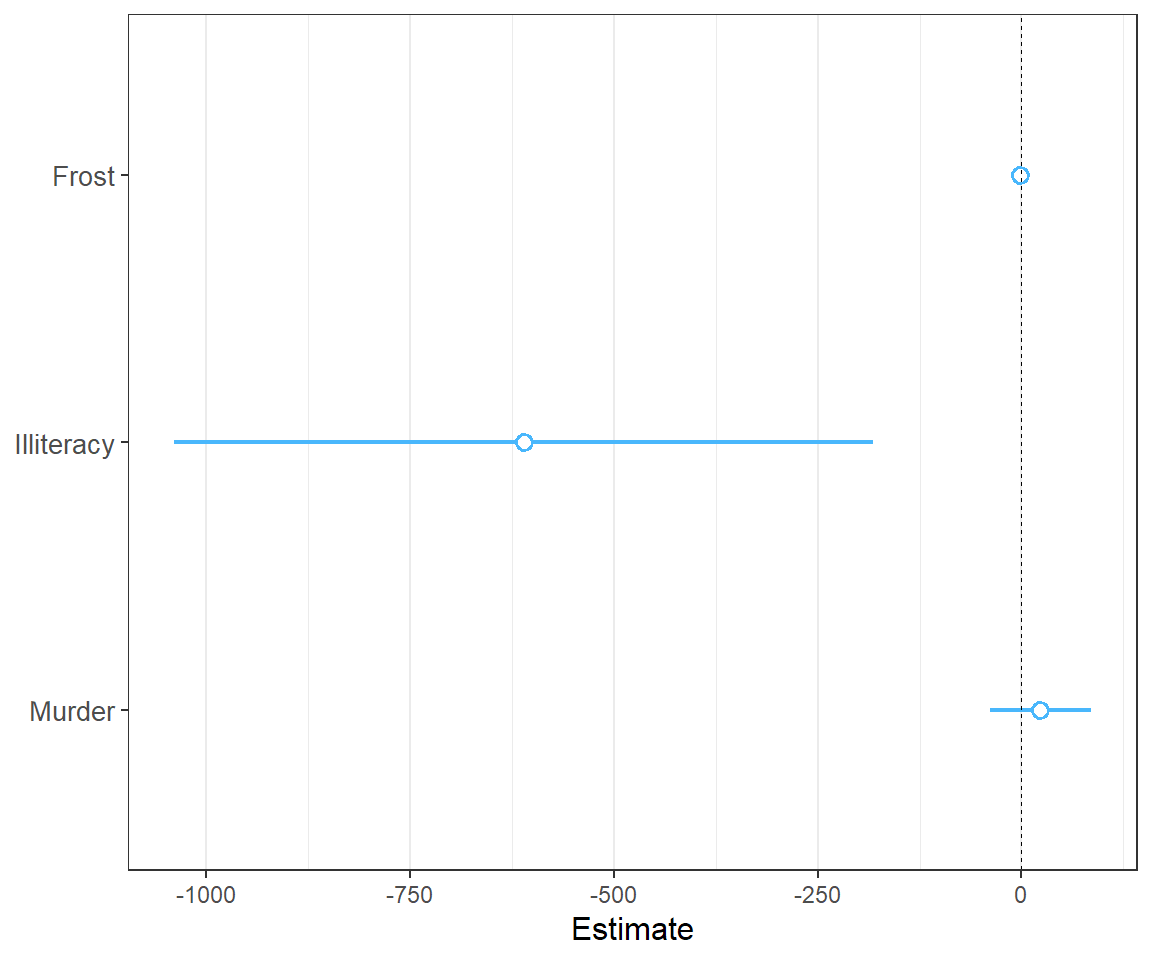Note that the intercept is omitted by default because it often distorts the scale and generally isn’t of theoretical interest. You can change this behavior or omit other coefficients with the `omit.coefs` argument.

In the above example, the differing scales of the variables makes it kind of difficult to make a quick assessment. No problem, we can just use the tools built into `summ` for that.

``plot_summs(fit, scale = TRUE)``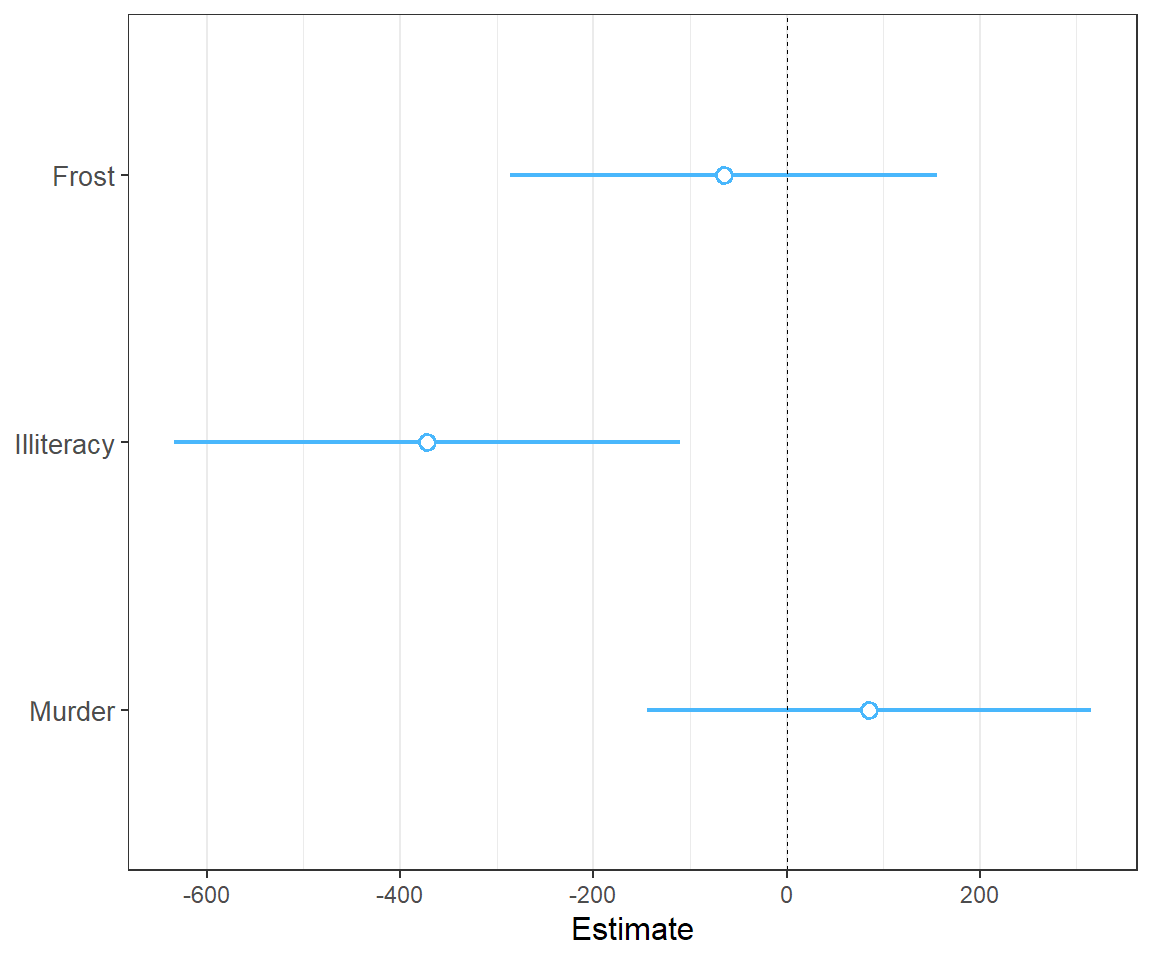See? Now we have a better idea of how the uncertainty and magnitude of effect differs for these variables. Note that by default the width of the confidence interval is .95, but this can be changed with the `ci_level` argument. You can also add a thicker band to convey a narrow interval using the `inner_ci_level` argument:

``plot_summs(fit, scale = TRUE, inner_ci_level = .9)``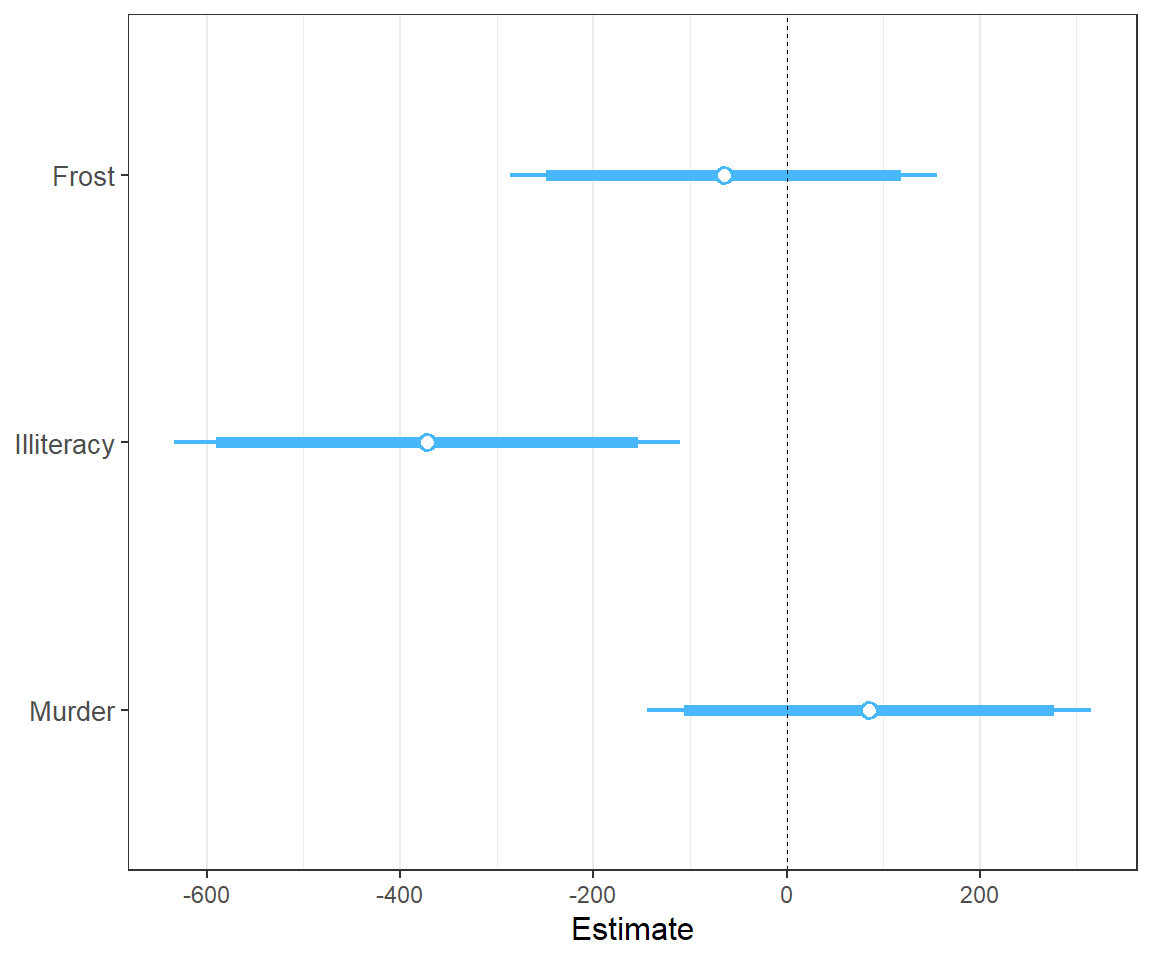Another compelling use case for `plot_summs` is robust standard errors ( just use the `robust` argument).

### Plot coefficient uncertainty as normal distributions

Most of our commonly used regression models make an assumption that the coefficient estimates are asymptotically normally distributed, which is how we derive our confidence intervals, p values, and so on. Using the `plot.distributions = TRUE` argument, you can plot a normal distribution along the width of your specified interval to convey the uncertainty. This is also great for didactic purposes.

While the common OLS model assumes a t distribution, I decided that they are visually sufficiently close that I have opted not to try to plot the points along a t distribution.

``plot_summs(fit, scale = TRUE, plot.distributions = TRUE, inner_ci_level = .9)``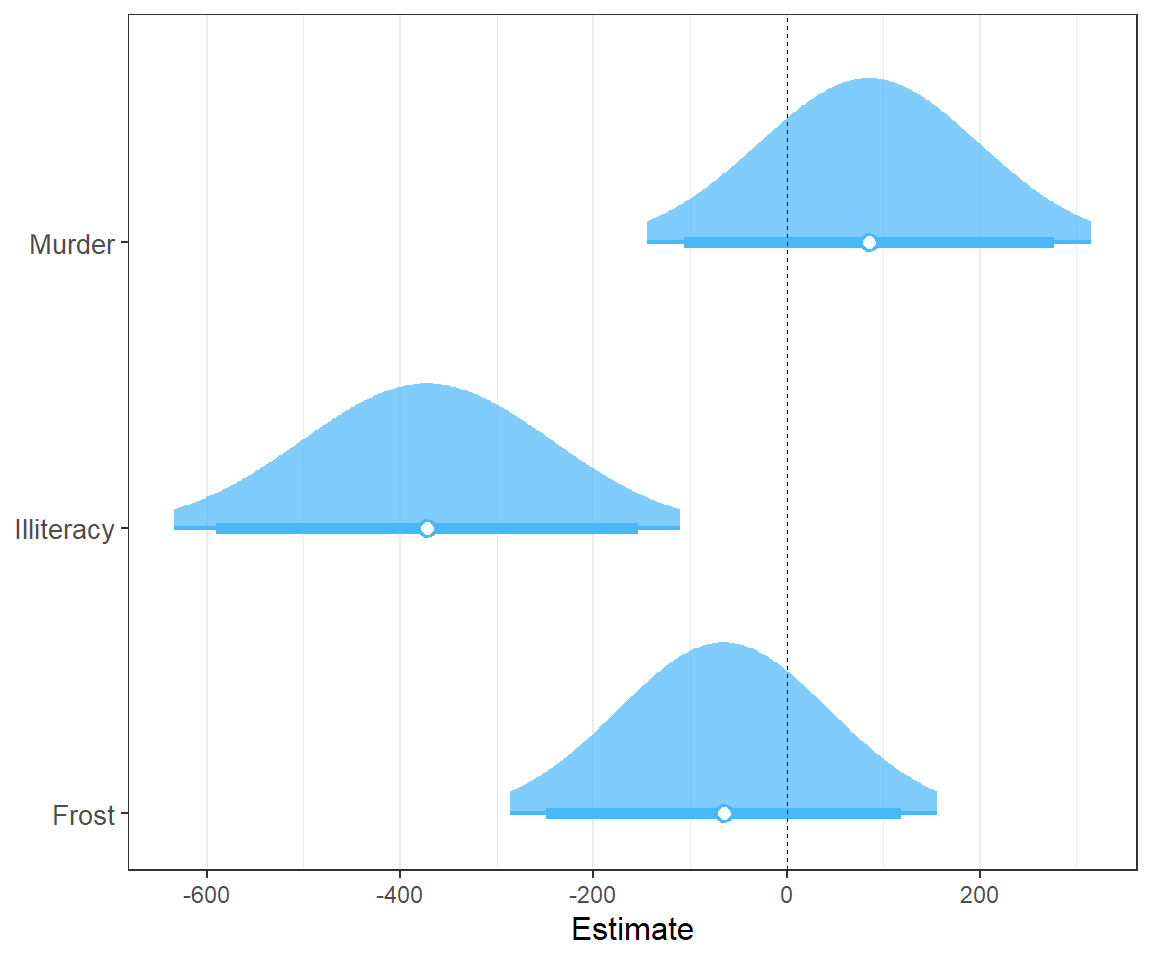### Comparing model coefficients visually

Comparison of multiple models simultaneously is another benefit of plotting. This is especially true when the models are nested. Let’s fit a second model and compare.

``````fit2 <- lm(Income ~ Frost + Illiteracy + Murder + `HS Grad`,
data = states)
plot_summs(fit, fit2, scale = TRUE)``````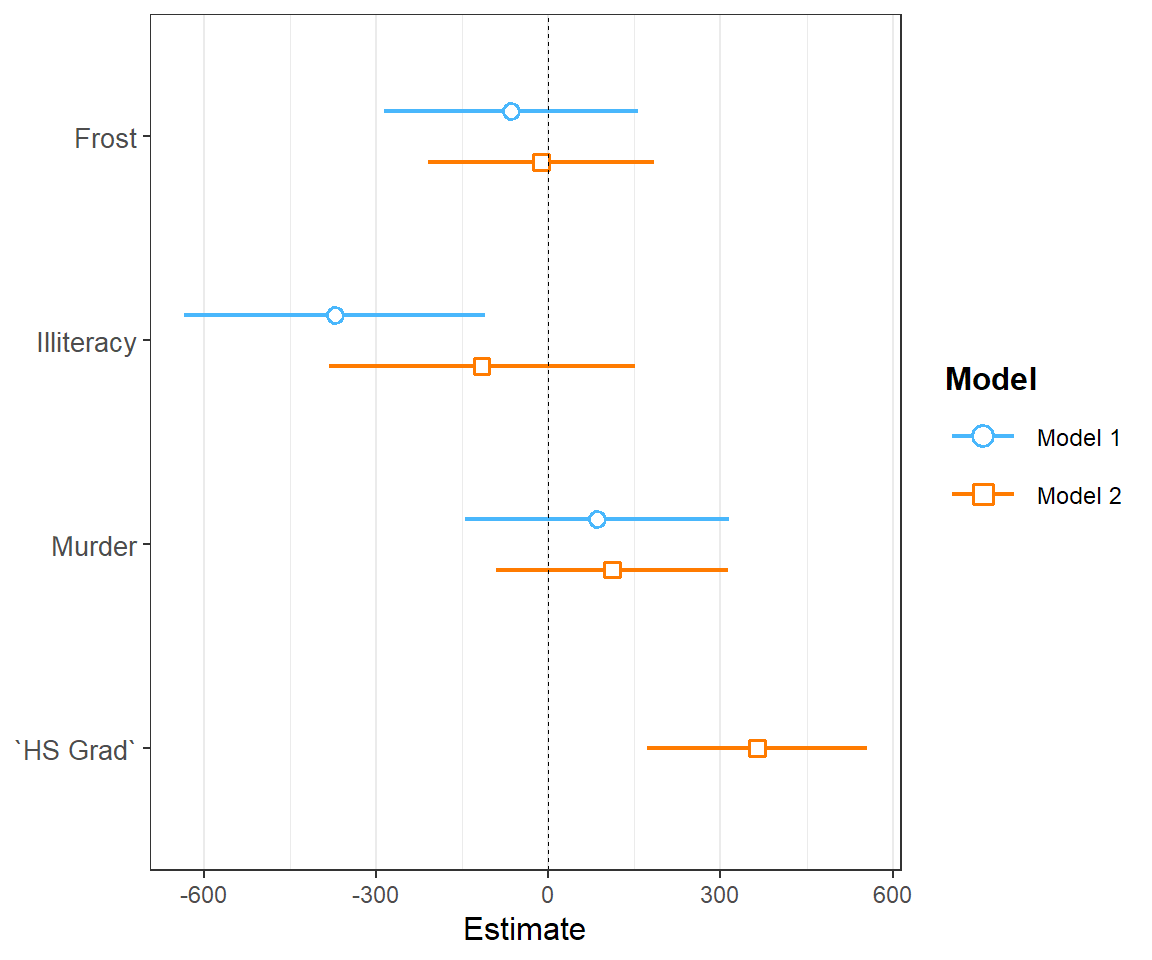This is a classic case in which adding a new predictor causes another one’s estimate to get much closer to zero.

Doing this with `plot.distributions = TRUE` creates a nice effect:

``plot_summs(fit, fit2, scale = TRUE, plot.distributions = TRUE)``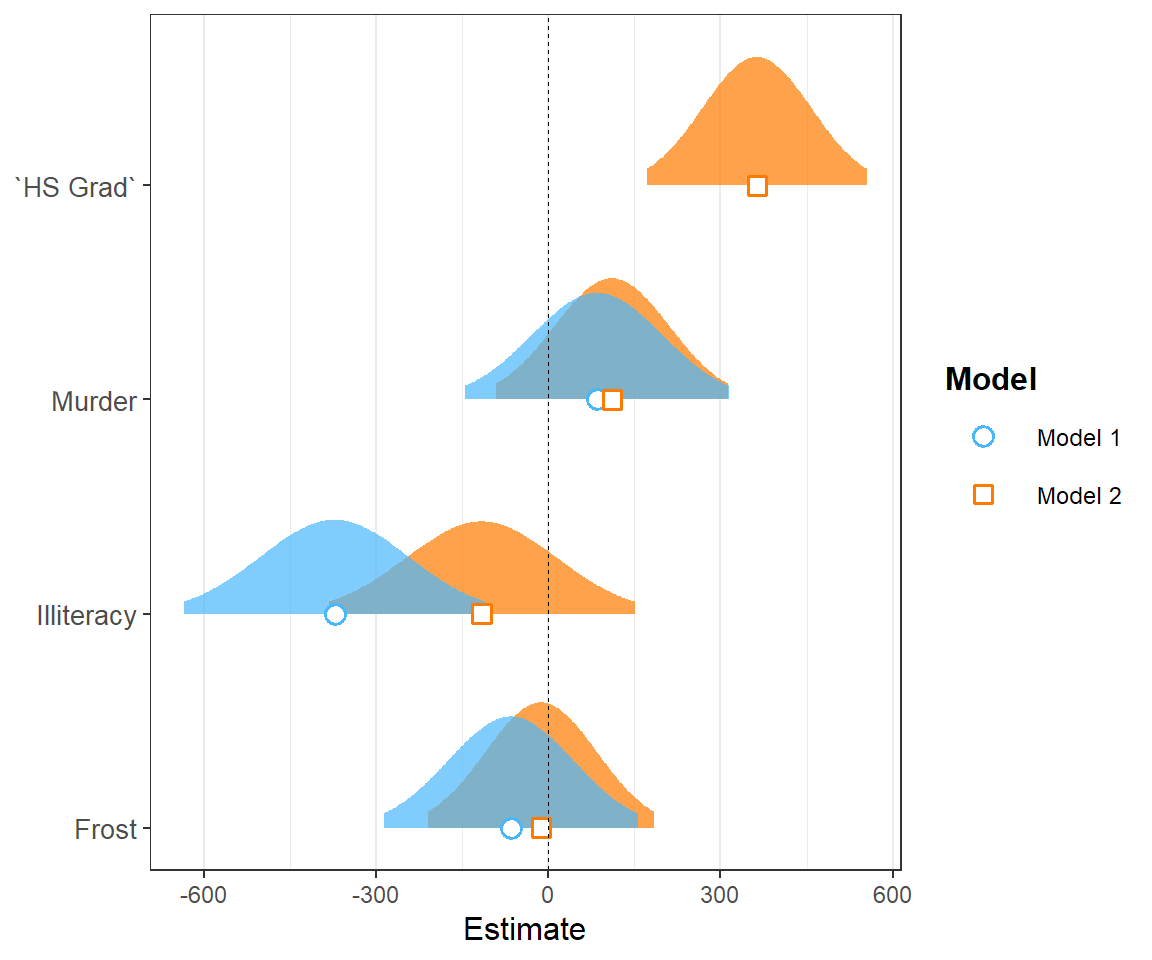By providing a list to `summ` arguments in `plot_summs`, you can compare results with different `summ` arguments (each item in the list corresponds to one model; the second list item to the second model, etc.). For instance, we can look at how the standard errors differ with different `robust` arguments:

``````plot_summs(fit, fit, fit, scale = TRUE, robust = list(FALSE, "HC0", "HC3"),
model.names = c("OLS", "HC0", "HC3"))``````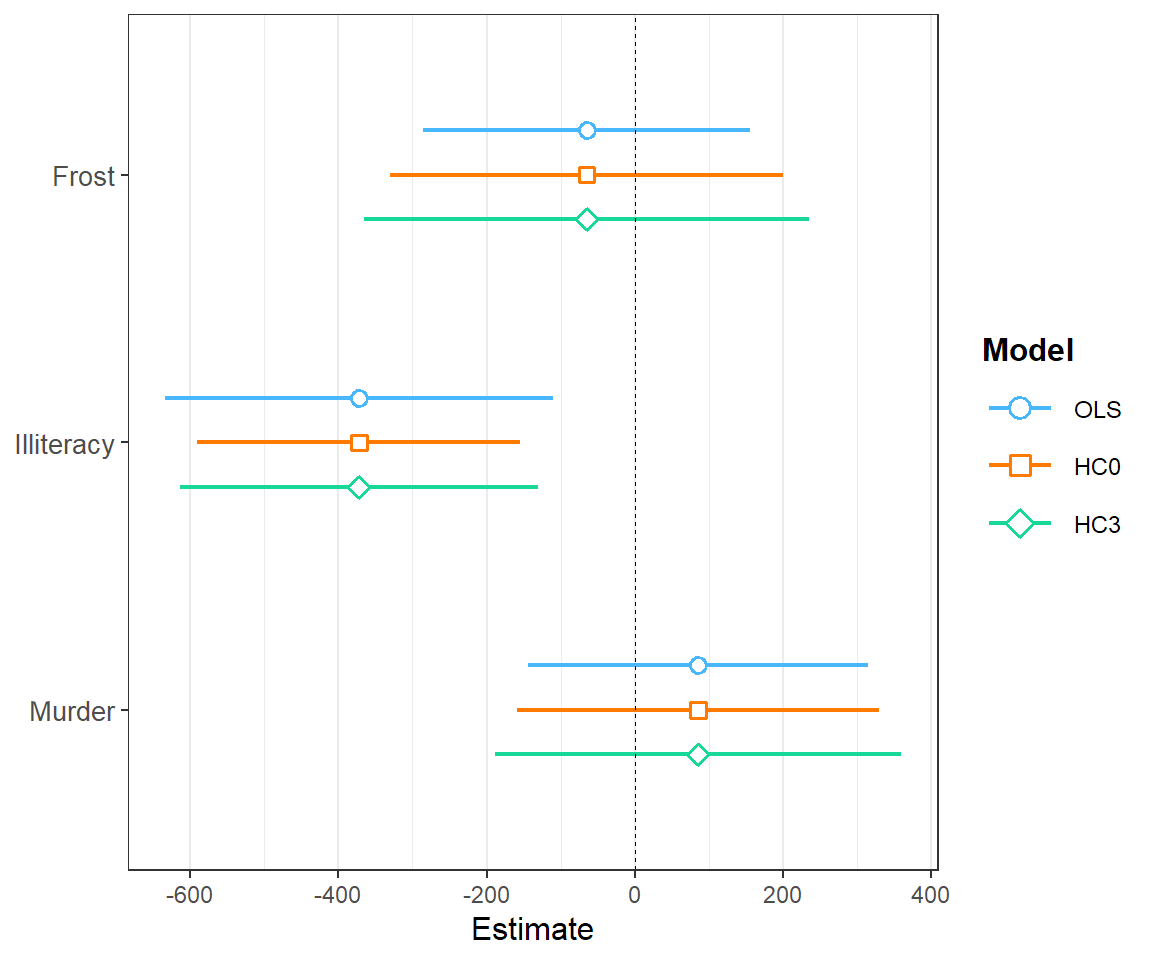`plot_coefs` is very similar to `plot_summs`, but does not offer the features that `summ` does. The tradeoff, though, is that it allows for model types that `summ` does not — any model supported by `tidy` from the `broom` package should work. If you provide unsupported model types to `plot_summs`, it just passes them to `plot_coefs`.

## `effect_plot`

Sometimes to really understand what your model is telling you, you need to see the kind of predictions it will give you. For that, you can use `effect_plot`, which is similar to `interact_plot` (see other vignette) but for main effects in particular.

Here’s the most basic use with our linear model:

``effect_plot(fit, pred = Illiteracy)``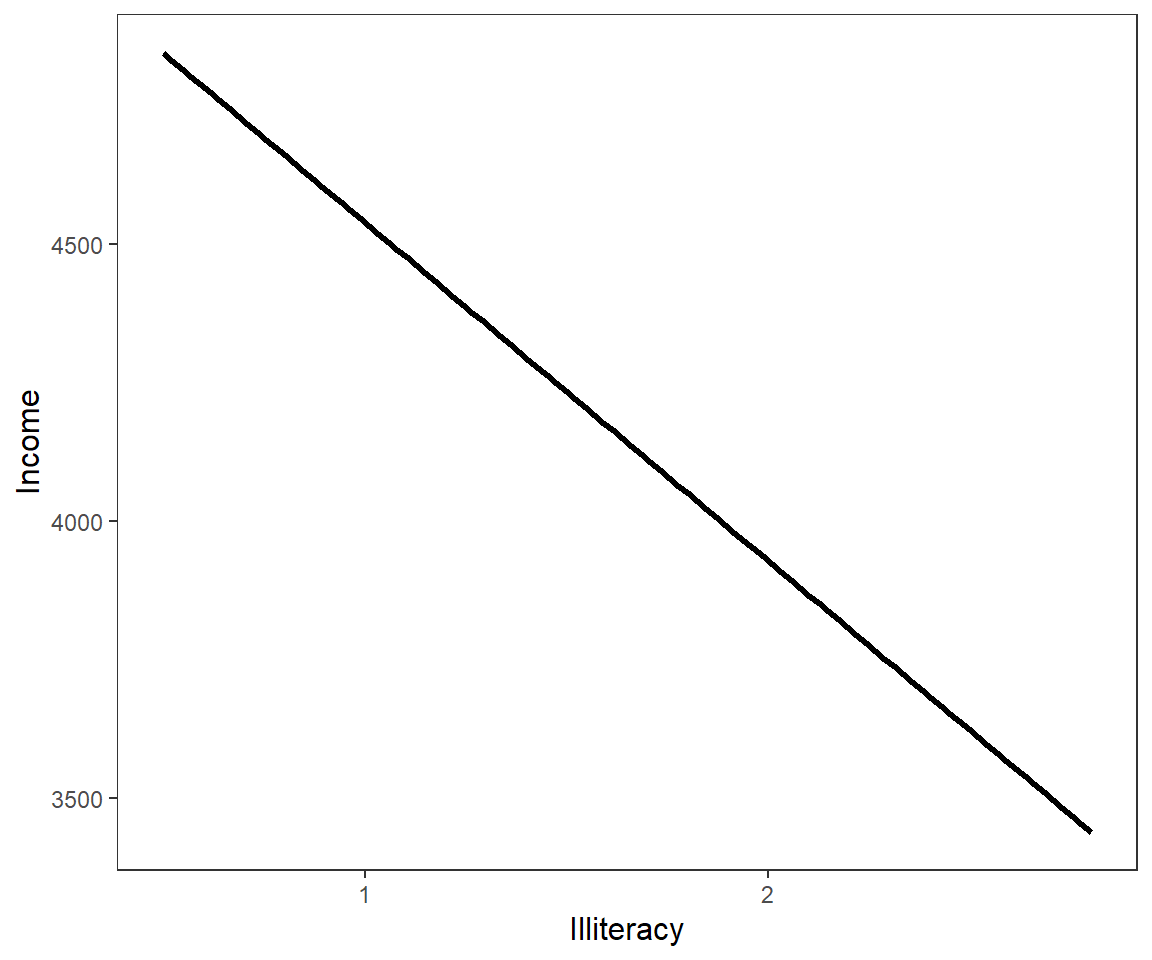Okay, not so illuminating. Let’s see the uncertainty around this line.

``effect_plot(fit, pred = Illiteracy, interval = TRUE)``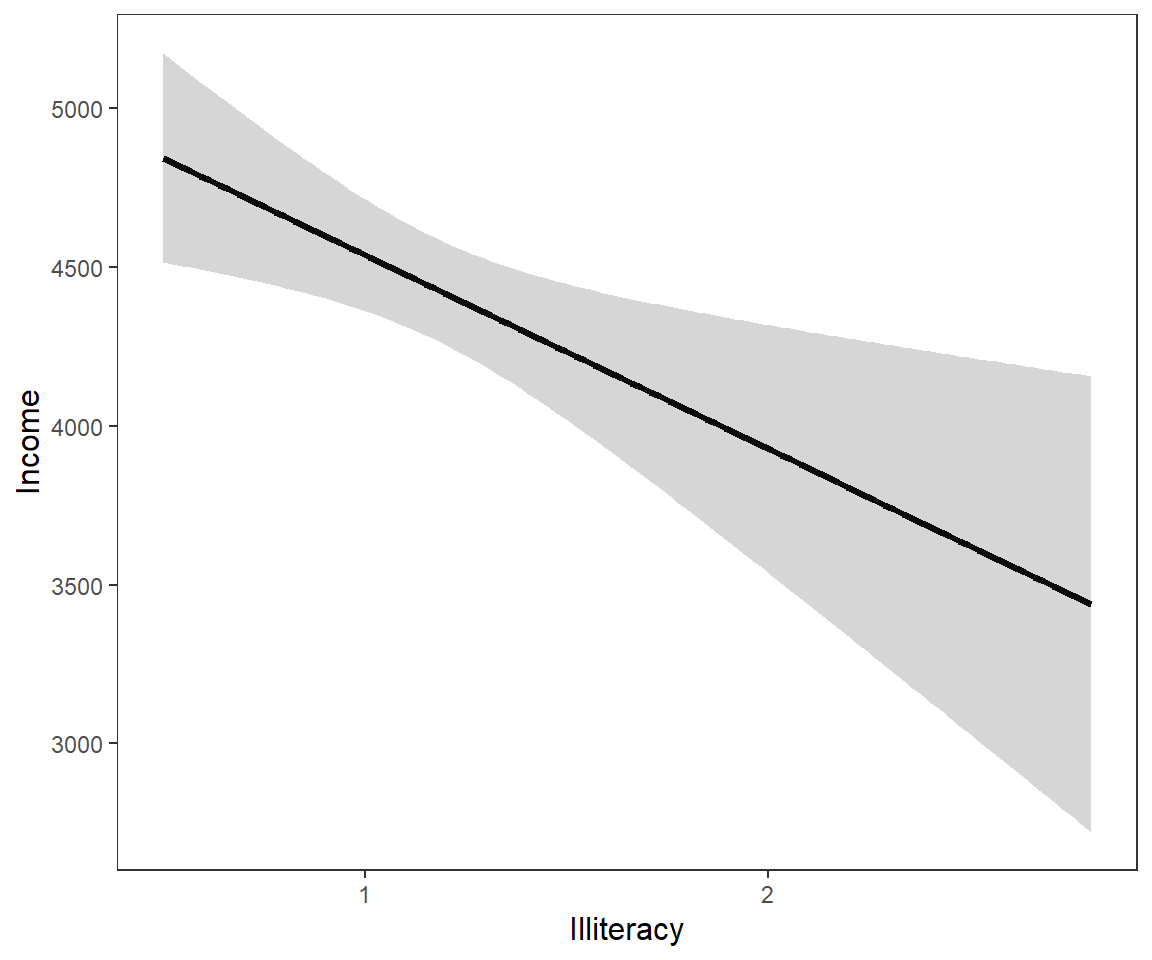Now we’re getting somewhere.

How does this compare to the observed data? Let’s see!

``effect_plot(fit, pred = Illiteracy, interval = TRUE, plot.points = TRUE)``